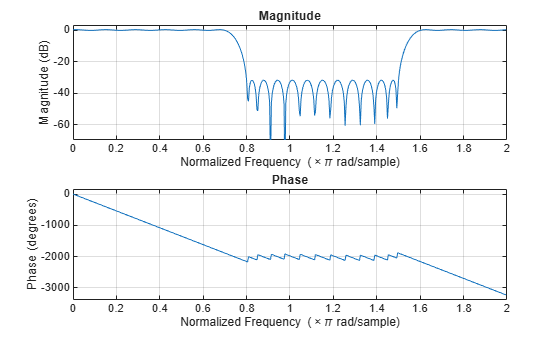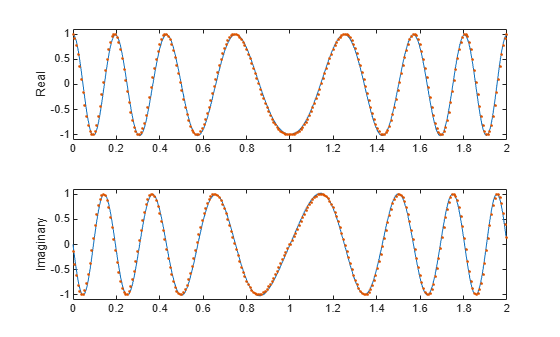# cfirpm

Complex and nonlinear-phase equiripple FIR filter design

## Syntax

``b = cfirpm(n,f,fresp)``
``b = cfirpm(n,f,fresp,w)``
``b = cfirpm(n,f,a)``
``b = cfirpm(n,f,a,w)``
``b = cfirpm(___,sym)``
``b = cfirpm(___,debug)``
``b = cfirpm(___,lgrid)``
``b = cfirpm(___,'skip_stage2')``
``[b,delta] = cfirpm(___)``
``[b,delta,opt] = cfirpm(___)``

## Description

example

````b = cfirpm(n,f,fresp)` returns a length `n`+1 FIR filter with the best approximation to the desired frequency response as returned by the `fresp` function, which is called by its function handle (`@fresp`).```
````b = cfirpm(n,f,fresp,w)` uses the weights specified by `w` to weight the fit in each frequency band.```
````b = cfirpm(n,f,a)` specifies amplitudes `a` at the band edges in `f`. This syntax returns the same result as ```b = cfirpm(n,f,{@multiband,a})```.```
````b = cfirpm(n,f,a,w)` applies an optional set of positive weights, one per band, for use during optimization. If you do not specify `w`, the function sets the weights to unity.```

example

````b = cfirpm(___,sym)` imposes a symmetry constraint on the impulse response of the design. In addition to specifying `sym`, specify an input combination from any of the previous syntaxes.```
````b = cfirpm(___,debug)` displays or hides the intermediate results during the filter design.```
````b = cfirpm(___,lgrid)` controls the density of the frequency grid.```
````b = cfirpm(___,'skip_stage2')` disables the second-stage optimization algorithm, which executes only when the `cfirpm` function determines that an optimal solution has not been reached by the standard `firpm` error-exchange. Disabling this algorithm can increase the speed of computation but incur a reduction in accuracy. By default, the second-stage optimization is enabled.```

example

````[b,delta] = cfirpm(___)` returns the maximum ripple height `delta`.```
````[b,delta,opt] = cfirpm(___)` returns optional results computed by the `cfirpm` function.```

## Examples

collapse all

Design a 31-tap linear-phase lowpass filter. Display its magnitude and phase responses.

```b = cfirpm(30,[-1 -0.5 -0.4 0.7 0.8 1],@lowpass); fvtool(b,1,'OverlayedAnalysis','phase')```Design a nonlinear-phase allpass FIR filter of order 22 with frequency response given approximately by $\mathrm{exp}\left(-j\pi fN/2+j4\pi f|f|\right)$, where $f\in \left[-1,1\right]$.

```n = 22; % Filter order f = [-1 1]; % Frequency band edges w = [1 1]; % Weights for optimization gf = linspace(-1,1,256); % Grid of frequency points d = exp(-1i*pi*gf*n/2 + 1i*pi*pi*sign(gf).*gf.*gf*(4/pi)); % Desired frequency response```

Use `cfirpm` to compute the FIR filter. Plot the actual and approximate magnitude responses in dB and the phase responses in degrees.

```b = cfirpm(n,f,'allpass',w,'real'); % Approximation freqz(b,1,256,'whole') subplot(2,1,1) % Overlay response hold on plot(pi*(gf+1),20*log10(abs(fftshift(d))),'r--') subplot(2,1,2) hold on plot(pi*(gf+1),unwrap(angle(fftshift(d)))*180/pi,'r--') legend('Approximation','Desired','Location','SouthWest')```Design a lowpass filter of order 30 using a custom frequency response function `fresp`. The code for the `fresp` function is available at the end of the example.

`[b,delta]= cfirpm(30,linspace(-1,1,32),@fresp);`

Use `FVTool` to visualize the magnitude response of the filter.

`fvtool(b,1)`User-Defined `fresp` Function: Design a lowpass filter

The `fresp` function lets you choose to design a lowpass filter, a highpass filter, or a differentiator. The filter order `N` and frequency array `F` must be specified. If the frequency grid `GF` and weights `W` are unspecified, the function determines those values automatically.

```function [dh,dw] = fresp(N,F,GF,W) W = [1;1]*(W(:).'); W = W(:); type = 'lowpass'; mags = zeros(size(W)); switch type case 'lowpass' mags(10:end-10) = 1; case 'highpass' mags(1:10) = 1; mags(end-10:end) = 1; case 'differentiator' mags = abs(linspace(-pi,pi,length(mags))); end dh = interp1(F(:),mags,GF).*exp(-1j*pi*GF*N/2); dw = interp1(F(:),W,GF); end```

## Input Arguments

collapse all

Filter order, specified as a real positive scalar.

Normalized frequency points, specified as a real-valued vector with elements in the range [–1, 1], where 1 corresponds to the normalized Nyquist frequency. The frequencies must be in increasing order, and `f` must have even length. The frequency bands span `f(k)` to `f`(k+1) for k odd. The intervals `f`(k+1) to `f`(k+2) for k odd are transition bands or don't care regions during optimization.

Frequency response, specified as a function handle. For more information, see Predefined Frequency Response Functions and User-Defined Frequency Response Functions.

Desired amplitudes at the points specified in `f`, specified as a vector. The desired amplitude at frequencies between pairs of points `f`(k) and `f`(k+1) for k odd is the line segment connecting the points (`f`(k),`a`(k)) and (`f`(k+1),`a`(k+1)).

Weights used to adjust the fit in each frequency band, specified as a real-valued vector. The length of `w` is half the length of `f`, so exactly one weight exists per band. If you do not specify `w`, the function sets the weights to unity.

Symmetry constraint imposed on the impulse response of the filter design, specified as one of these values:

• `'none'` — Impose no symmetry constraint. This option is the default if you pass any negative band frequencies to the function or if `fresp` does not supply a default value.

• `'even'` — Impose a real and even impulse response. This option is the default for highpass, lowpass, allpass, bandpass, bandstop, inverse-sinc, and multiband designs.

• `'odd'` — Impose a real and odd impulse response. This option is the default for Hilbert and differentiator designs.

• `'real'` — Impose conjugate symmetry for the frequency response.

If you specify a value other than `'none'`, you must specify the band edges over only positive frequencies (the negative frequency region is filled in from symmetry). If you do not specify `sym`, the function queries `fresp` for a default setting. Any user-supplied `fresp` function must return a valid `sym` option when it is passed `'defaults'` as the filter order `n`.

Display of intermediate results during the filter design, specified as `'off'`, `'trace'`, `'plots'`, or `'both'`.

Density of frequency grid, specified as a cell array of an integer. The frequency grid has roughly `2^nextpow2(lgrid*n)` frequency points.

## Output Arguments

collapse all

Filter coefficients, returned as a row vector of length `n`+1.

Maximum ripple height, returned as a scalar.

Optional results computed by the `cfirpm` function, returned as a structure containing these fields.

Field

Description

`opt.fgrid`

Frequency grid vector used for the filter design optimization

`opt.des`

Desired frequency response for each point in `opt.fgrid`

`opt.wt`

Weighting for each point in `opt.fgrid`

`opt.H`

Actual frequency response for each point in `opt.fgrid`

`opt.error`

Error at each point in `opt.fgrid`

`opt.iextr`

Vector of indices into `opt.fgrid` for extremal frequencies

`opt.fextr`

Vector of extremal frequencies

collapse all

### Predefined Frequency Response Functions

Predefined `fresp` frequency response functions are included for a number of common filter designs in this section. For more information on how to create a custom `fresp` function, see Create Function Handle.

For all of the predefined frequency response functions, the symmetry option `sym` defaults to `'even'` if `f` contains no negative frequencies and `d` = `0`. Otherwise `sym` defaults to `'none'`. For details, see `sym`. For all of the predefined frequency response functions, `d` specifies a group-delay offset such that the filter response has a group delay of `n`/2+`d` in units of the sample interval. Negative values create less delay, and positive values create more delay. By default, `d` = `0`.

• `@lowpass`, `@highpass`, `@allpass`, `@bandpass`, `@bandstop`

These functions share a common syntax, exemplified by `@lowpass`.

`b = cfirpm(n,f,@lowpass,...)` and

`b = cfirpm(n,f,{@lowpass,d},...)` design a linear-phase (`n`/2+`d` delay) filter.

Note

For `@bandpass` filters, the first element in the frequency vector must be less than or equal to zero and the last element must be greater than or equal to zero.

• `@multiband` designs a linear-phase frequency response filter with arbitrary band amplitudes.

`b = cfirpm(n,f,{@multiband,a},...)` and

`b = cfirpm(n,f,{@multiband,a,d},...)` specify vector `a` containing the desired amplitudes at the band edges in `f`. The desired amplitude at frequencies between pairs of points `f`(k) and `f`(k+1) for k odd is the line segment connecting the points (`f`(k),`a`(k)) and (`f`(k+1),`a`(k+1)).

• `@differentiator` designs a linear-phase differentiator. For these designs, zero-frequency must be in a transition band, and band weighting is set to be inversely proportional to frequency.

`b = cfirpm(n,f,{@differentiator,fs},...)` and

`b = cfirpm(n,f,{@differentiator,fs,d},...)` specify the sample rate `fs` used to determine the slope of the differentiator response. If omitted, `fs` defaults to 1.

• `@hilbfilt` designs a linear-phase Hilbert transform filter response. For Hilbert designs, zero-frequency must be in a transition band.

`b = cfirpm(n,f,@hilbfilt,...)` and

`b = cfirpm(N,F,{@hilbfilt,d},...)` design a linear-phase (`n`/2+`d` delay) Hilbert transform filter.

• `@invsinc` designs a linear-phase inverse-sinc filter response.

`b = cfirpm(n,f,{@invsinc,a},...)` and

`b = cfirpm(n,f,{@invsinc,a,d},...)` specify gain `a` for the sinc function, computed as sinc(`a`*g), where g contains the optimization grid frequencies normalized to the range [–1, 1]. By default, `a` = 1. The group-delay offset is `d` such that the filter response has a group delay of n/2+`d` in units of the sample interval, where n is the filter order. Negative values create less delay, and positive values create more delay. By default, `d` = 0.

### User-Defined Frequency Response Functions

Instead of the predefined frequency response functions for `fresp`, you can use a user-defined function.

The `cfirpm` function calls this user-defined function using this syntax.

```[dh,dw] = fresp(n,f,gf,w,p1,p2,...) ```

• `n` is the filter order.

• `f` is the vector of frequency band edges that appear monotonically between –1 and 1, where 1 corresponds to the Nyquist frequency.

• `gf` is a vector of grid points that have been linearly interpolated over each specified frequency band by `cfirpm`. The input `gf` determines the frequency grid at which the response function must be evaluated. The `cfirpm` function returns this data in the `fgrid` field of the `opt` structure.

• `w` is a vector of real, positive weights, one per band, used during optimization. `w` is optional in the call to `cfirpm`. If you do not specify this input, `cfirpm` sets it to unity weighting before passing it to `fresp`.

• `dh` and `dw` are the desired complex frequency response and band weight vectors, respectively, that are evaluated at each frequency in grid `gf`.

• `p1,p2,...` are optional parameters that can be passed to `fresp`.

Additionally, the `cfirpm` function makes a preliminary call to `fresp` to determine the default symmetry `sym`. `cfirpm` makes this call using this syntax.

```sym = fresp('defaults',{n,f,[],w,p1,p2,...}) ```
The arguments can be used in determining an appropriate symmetry default as necessary. You can use the local function `lowpass` as a template for generating new frequency response functions. To find the `lowpass` function, enter ```edit cfirpm``` at the command line and search for `lowpass` in the `cfirpm` function code. You can copy the function, modify it, rename it, and save it in your path.

## Algorithms

The `cfirpm` function enables you to specify arbitrary frequency-domain constraints for the design of a possibly complex FIR filter. The Chebyshev (or minimax) filter error is optimized, producing equiripple FIR filter designs.

An extended version of the Remez exchange method is implemented for the complex case. This exchange method obtains the optimal filter when the equiripple nature of the filter is restricted to have `n`+2 extremals. When the filter does not converge, the algorithm switches to an ascent-descent algorithm that takes over to finish the convergence to the optimal solution. For further details, see the references.

 Demjanjov, V. F., and V. N. Malozemov. Introduction to Minimax. New York: John Wiley & Sons, 1974.

 Karam, L.J. Design of Complex Digital FIR Filters in the Chebyshev Sense. Ph.D. Thesis, Georgia Institute of Technology, March 1995.

 Karam, L.J., and J. H. McClellan. "Complex Chebyshev Approximation for FIR Filter Design." IEEE® Transactions on Circuits and Systems II: Analog and Digital Signal Processing 42, no. 3 (March 1995): 207–216.